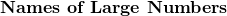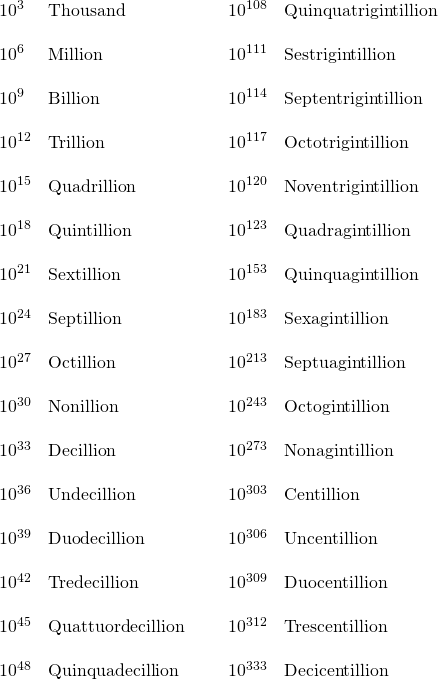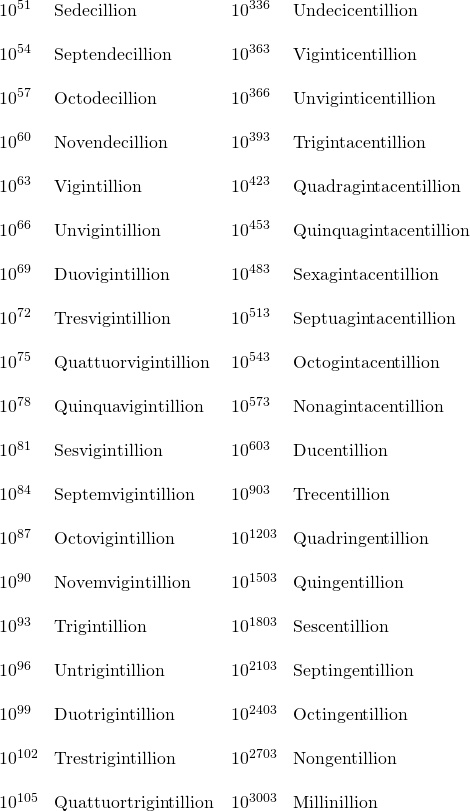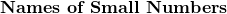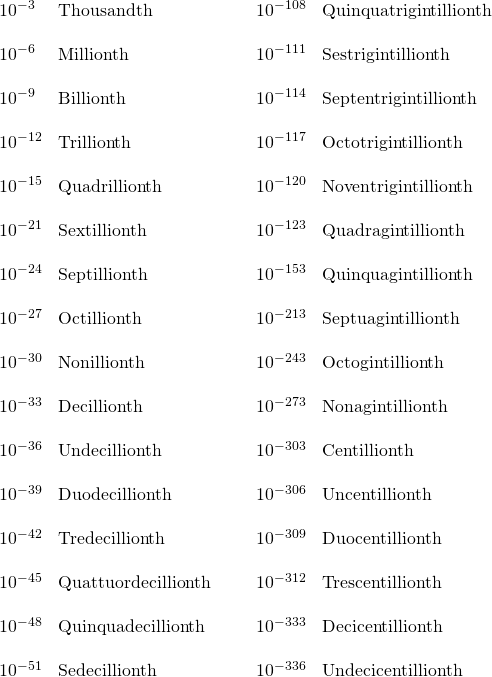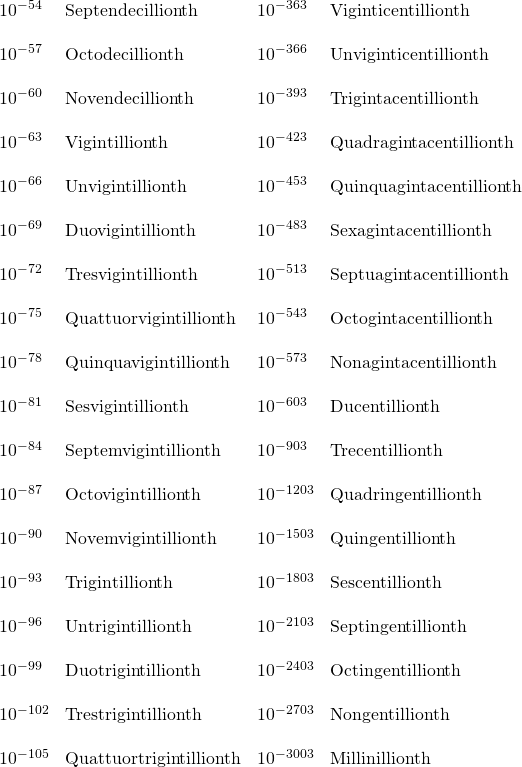Chapter 1: Algebra Review

# 1.5 Terms and Definitions

Digits can be defined as the alphabet of the Hindu–Arabic numeral system that is in common usage today. This alphabet is: 0, 1, 2, 3, 4, 5, 6, 7, 8, 9. Written in set-builder notation, digits are expressed as: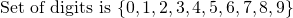Natural numbers are often called counting numbers and are usually denoted by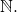These numbers start at 1 and carry on to infinity, which is denoted by the symbol ∞. Writing the set of natural numbers in set-builder notation gives: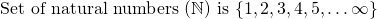Whole numbers include the set of natural numbers and zero. Whole numbers are generally designated by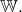In set-builder notation, the set of whole numbers is denoted by: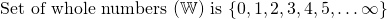Integers include the set of all whole numbers and their negatives. This means the set of integers is composed of positive whole numbers, negative whole numbers, and zero (fractions and decimals are not integers). Common symbols used to represent integers are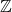and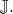For this textbook, the symbolwill be used to represent integers.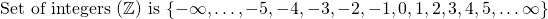Rational numbers include all integers and all fractions, terminating decimals, and repeating decimals. Every rational number can be written as a fraction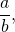where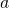and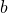are integers. Rational numbers are denoted by the symbol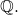In set-builder notation, the set of rational numbers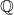can be informally written as: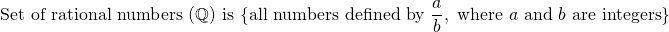Irrational numbers include any number that cannot be defined by the fractionwhereandare integers. These are numbers that are non-repeating or non-terminating. Classic examples of irrational numbers are pi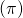and the square roots of 2 and 3. The symbol for irrational numbers is commonly given asor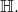For this textbook, the symbolwill be used. In set-builder notation, the set of irrational numberscan be informally written as: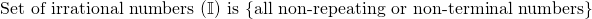Real numbers include the set of all rational numbers and irrational numbers. The symbol for real numbers is commonly given as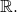In set-builder notation, the set of real numbers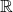can be informally written as: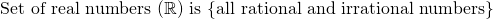Numbers that may not yet have been encountered are imaginary numbers (commonly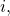sometimes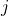) and complex numbers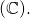These numbers will be properly defined later in the textbook.

Imaginary numbers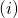include any real number multiplied by the square root of −1.

Complex numbers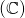are combinations of any real number, imaginary number, or a sum and difference of them.

Consecutive integers are integers that follow each other sequentially. Examples are: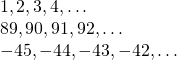Consecutive even or odd integers are numbers that skip the odd/even sequence to just show odd, odd, odd, or even, even, even. Examples are: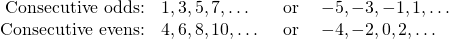Prime numbers are numbers that cannot be divided by any integer other than 1 and itself. The following is a list of all the prime numbers that are found between 0 and 1000. (Note: 1 is not considered prime.)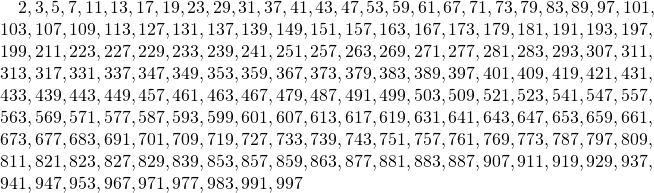Squares are numbers multiplied by themselves. A number that is being squared is shown as having a superscript 2 attached to it. For example, 5 squared is written as 52, which equals 5 × 5 or 25.

Perfect squares are squares of whole numbers, such as 1, 4, 9, 16, 25, 36, and 49. They are found by squaring natural numbers. The following is the list of perfect squares using numbers up to 20: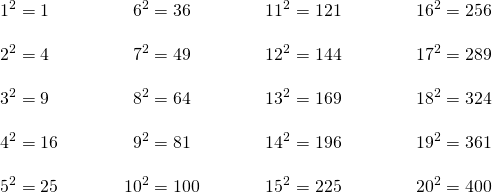Cubes are numbers multiplied by themselves three times. A number that is being cubed is shown as having a superscript 3 attached to it. For example, 5 cubed is written as 53, which equals 5 × 5 × 5 or 125.

Perfect cubes are cubes of whole numbers, such as 1, 8, 27, 64, 125, 216, and 343. They are found by cubing natural numbers. The following is the list of perfect cubes using numbers up to 20: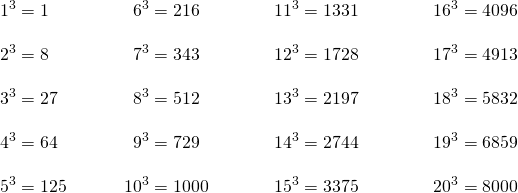Percentage means parts per hundred. A percentage can be thought of as a fractionwhere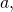the numerator, is the number to the left of the % sign, and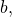the denominator, is 100. For example: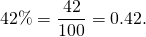Absolute values. The absolute value of an expressiondenoted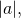is the distance from zero of the number or operation that occurs between the absolute value signs. For example: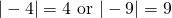Examples of absolute values of simple operations are: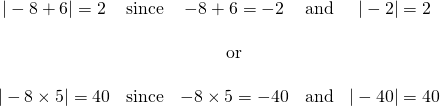Set-builder notation follows standard patterns and is as follows:

• Begin the set with a left brace {
• A vertical bar | means “such that”
• End the set with a right brace }

So to say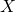is an integer, write this as: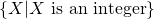This means “the set of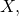such thatis an integer.”

Another way of writing this is to use the symbols that mean “element of” and “not an element of.”

“Element of” is shown by the symbol ∈, and “not an element of” is shown by the element symbol with a line drawn through it, ∉.

In simplest terms, if something is an element of something else, it means that it belongs to or is part of it. For example, a set of numbers called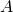can only be made up of any natural number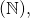like 4, 6, 9, and 15. This can be stated as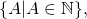which reads as “the set of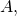such thatis an element of the natural number system.”

“Not an element of” can be used to state that the set cannot contain excluded values. For example, say there is a set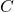of all numbersexcept counting numbersThis can be written as: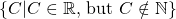This can be read as “the set of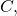such thatis an element of the set of all real numbers, excluding those numbers that are natural numbers.”

Sets of numbers giving excluded values can be seen throughout this textbook. The standard example is to exclude values that would result in a denominator of zero. This exclusion avoids division by zero and getting an undefinable answer.

The empty set. Sometimes, a set contains no elements. This set is termed the “empty set” or the “null set.” To represent this, write either { } or Ø.